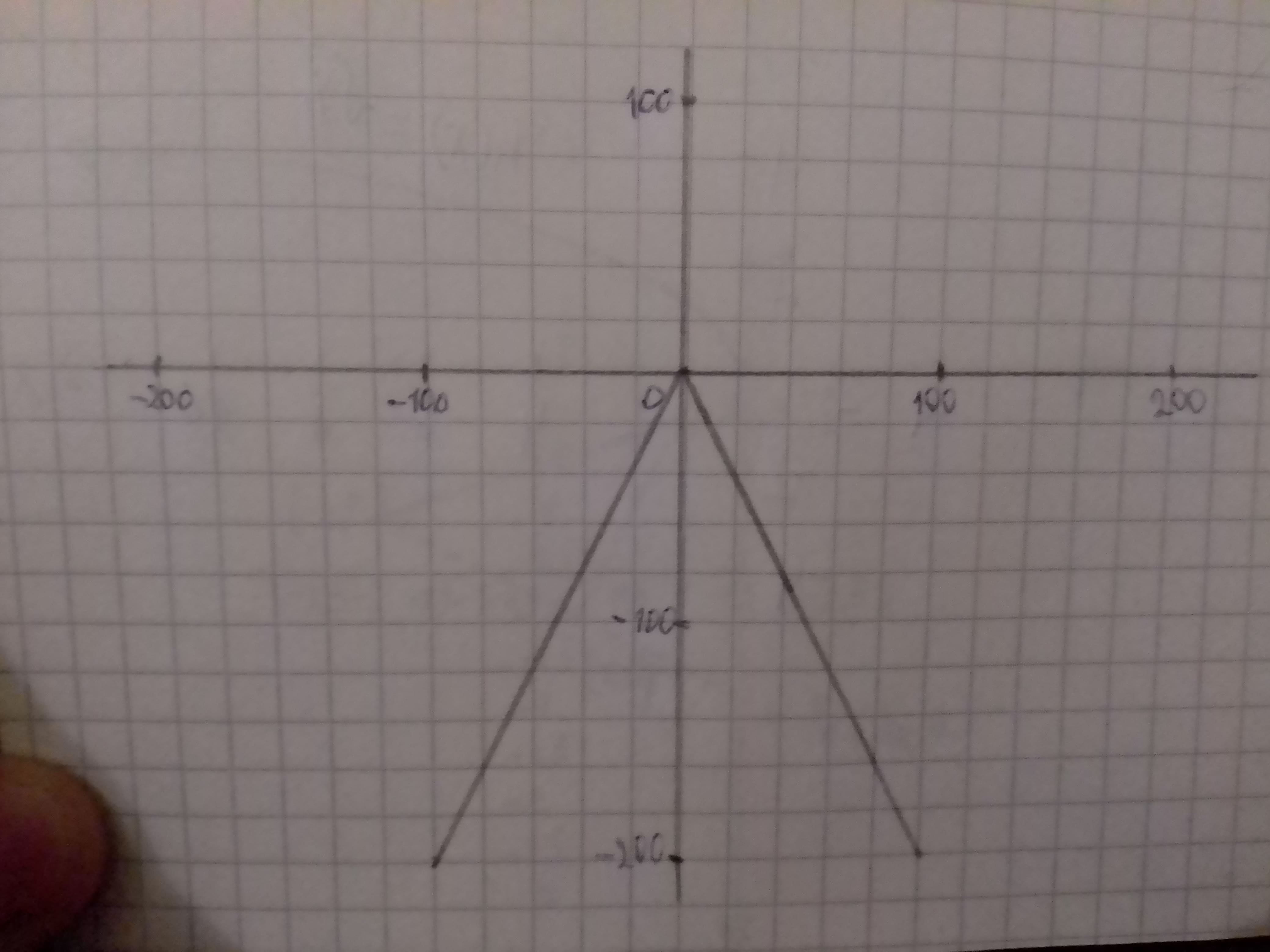# Provide answers to all tasks using the information provided. a) Find the parent function f. Given Information: g{{left({x}right)}}=-{2}{left|{x}-{1}rimelodykap 2021-02-25 Answered
a) Find the parent function f.
Given Information: $g\left(x\right)=-2|x-1|-4$
b) Find the sequence of transformation from f to g.
Given information: $f\left(x\right)=\left[x\right]$
c) To sketch the graph of g.
Given information: $g\left(x\right)=-2|x-1|-4$
d) To write g in terms of f.
Given information: $g\left(x\right)=-2|x-1|-4\phantom{\rule{1em}{0ex}}\text{and}\phantom{\rule{1em}{0ex}}f\left(x\right)=\left[x\right]$
You can still ask an expert for help

• Questions are typically answered in as fast as 30 minutes

Solve your problem for the price of one coffee

• Math expert for every subject
• Pay only if we can solve itGennenzip

a)
Parent function is the basic function of a family of functions that preserves the definitions, shape of its graph and properties of the entire family.
Parent function used in this question is the absolute value function $f\left(x\right)=\left[x\right]$
To identify the parent function, strip all the arithmetic operations on the function to leave behind one higher order operation in just x.
So, remove the arithmetic operation of multiplication by -1 and then addition of 4 to x and addition of 8 to it from the given funcion to get the parent function.
Conclusion:
So, remove the arithmetic operation of subtractionof 1 from x and multiplication by -2 and then subtraction of g

b)
The sequence of transformations from f to g depicts the steps followed and the transformations used to reach from the parent function f to g.
Types of shifts used in function transformation:
1. Vertical shift: If c is a real number which is also positive, then the graph of shifted upward by c units.
If c is a real number which is also positive, thenthe graph of shifted downwards by c units.
2. Horizontal Shift: If c is a real number which is also positive then, the graph of shifted left by c units.
If c is a real number which is also positive then, the graph of shifted right by c units.
3. Reflection: The graph for the function say is the reflection in y-axis.
The graph for the function say is the reflection in x-axis.
4. Vertical Stretching and Shrinking: If c succ 1 then, the graph of stretched vertically by c units.
If 0 prec c prec 1 then, the graph of shrunk vertically by c units.
5. Horizontal Stretching and Shrinking: If c succ 1 then, the graph of shrunk horizontally by c units.
If 0 prec c prec 1 then, the graph of stretched horizontally by c units.
The shape of $g\left(x\right)=-2|x-1|-4$ is drawn reflected in the x-axis and then shifted right by 1 unit and stretched by 2 units and then downward by 4 units.
The sequence of transformations from f to g depicts the steps followed and the transformations used to reach from the parent function f to g.
Conclusion:
The shape of is drawn reflected in the x-axis and then shifted right by 1 unit and stretched by 2 units and then shifted downward by 4 units is the required sequence of transfomations from f to g.
c)
Use the sequence of tranformation to plot the graph of the function.
Obtain the graph of $g\left(x\right)=-2|x-1|-4$d)
Multiply and then subtract from the parent function to get g(x) in terms of f(x).